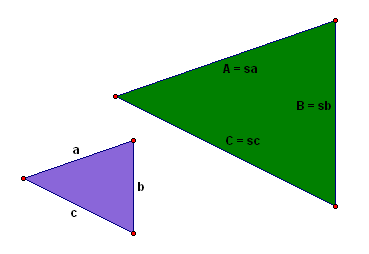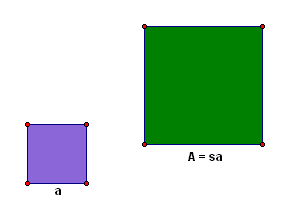﻿ Perimeters and Areas of Similar Polygons

#Perimeters and Areas of Similar Polygons

You may want to review: Similarity, Ratios, and Proportions

Suppose that a triangle with sides $\,a\,,$ $\,b\,$ and $\,c\,$ has been scaled by a positive number $\,s\,$ to get a similar triangle with corresponding sides $\,A\,,$ $\,B\,,$ and $\,C\,.$

Thus, $\,A = sa\,$ and $\,B = sb\,$ and $\,C = sc\,.$Let's investigate the relationship between the perimeters of these two triangles:

\begin{align} &\cssId{s8}{\text{perimeter of original triangle} = a + b + c}\cr\cr &\cssId{s9}{\text{perimeter of scaled triangle}}\cr &\qquad \cssId{s10}{= A + B + C}\cr &\qquad \cssId{s11}{= sa + sb + sc}\cr &\qquad \cssId{s12}{= s(a + b + c)}\cr &\qquad \cssId{s13}{= s(\text{perimeter of original triangle})}\cr \end{align}

Thus, the perimeter ends up being scaled by the same factor that scales the sides!

This can be re-phrased in terms of ratios. Notice that:

\begin{align} &\cssId{s17}{\frac{\text{scaled perimeter}}{\text{original perimeter}}}\cr\cr &\qquad \cssId{s18}{= \frac{A+B+C}{a+b+c}}\cr\cr &\qquad \cssId{s19}{= \frac{sa+sb+sc}{a+b+c}}\cr\cr &\qquad \cssId{s20}{= \frac{s(a+b+c)}{a+b+c}}\cr\cr &\qquad \cssId{s21}{= s}\cr\cr &\qquad \cssId{s22}{= \text{scaling factor}} \end{align}

and

$$\begin{gather} \cssId{s24}{\frac{\text{scaled side}}{\text{original side}}} \cssId{s25}{= \frac{A}{a}} \cssId{s26}{= \frac{sa}{a}} \cssId{s27}{= s}\cr\cr \cssId{s28}{\frac{\text{scaled side}}{\text{original side}} = \frac{B}{b} = \frac{sb}{b} = s}\cr\cr \cssId{s29}{\frac{\text{scaled side}}{\text{original side}} = \frac{C}{c} = \frac{sc}{c} = s} \end{gather}$$

So, the ratio of the perimeters is the same as the ratio of corresponding sides.

A similar calculation shows that this result is indeed true for polygons in general:

THEOREM Perimeters of Similar Polygons
The ratio of the perimeters of two similar polygons is equal to the ratio of the corresponding sides.

That is:

\begin{align} &\cssId{s36}{\frac{\text{perimeter of scaled polygon}}{\text{perimeter of original polygon}}}\cr\cr &\qquad \cssId{s37}{= \text{the scaling factor}}\cr\cr &\qquad \cssId{s38}{= \frac{\text{scaled side}}{\text{original side}}} \end{align}

Area, on the other hand, behaves quite differently.

Suppose you have a square of side $\,a\,$ that has been scaled by $\,s\,$ to get a square of side $\,A\,.$ Thus, $\,A = sa\,.$Let's investigate the relationship between the areas of these two squares:

\begin{align} &\cssId{s45}{\text{area of original square}} \cssId{s46}{= a\cdot a} \cssId{s47}{= a^2}\cr\cr &\cssId{s48}{\text{area of scaled square}}\cr &\qquad \cssId{s49}{= A\cdot A}\cr &\qquad \cssId{s50}{= (sa)\cdot(sa)}\cr &\qquad \cssId{s51}{= s^2\cdot a^2}\cr &\qquad \cssId{s52}{= s^2(\text{area of original square})} \end{align}

Thus, the area ends up being scaled by the square of the factor that scales the sides!

Again, this can be re-phrased in terms of ratios. Notice that:

\begin{align} &\cssId{s56}{\frac{\text{scaled square area}}{\text{original square area}}}\cr\cr &\qquad \cssId{s57}{= \frac{A\cdot A}{a \cdot a}}\cr\cr &\qquad \cssId{s58}{= \frac{(sa)\cdot (sa)}{a\cdot a}}\cr\cr &\qquad \cssId{s59}{= \frac{s^2a^2}{a^2}}\cr\cr &\qquad \cssId{s60}{= s^2}\cr\cr &\qquad \cssId{s61}{= \text{the square of the ratio of corresponding sides}} \end{align}

This idea leads to the following theorem:

THEOREM Areas of Similar Polygons
The ratio of the areas of two similar polygons is equal to the square of the ratio of the corresponding sides.

## Examples

Question: Suppose that a triangle has sides of lengths $\,x\,,$ $\,y\,,$ and $\,z\,.$ The sides of the triangle are scaled by a factor of $\,5\,$ to get a similar triangle. What is a formula for the perimeter of the new triangle?
Solution: The perimeter is scaled by the same factor as the sides. The original perimeter is $\,x+y+z\,.$ The new (scaled) perimeter is $\,5(x+y+z)\,.$
Question: Suppose that the sides of a polygon are scaled by a factor of $\,3\,$ to get a similar polygon. This new polygon has area $\,198\,.$ What is the area of the original polygon?
Solution: The area is scaled by the square of the factor that scales the sides. Let $\,A\,$ denote the (unknown) original area. We are told that the new (scaled) area is $\,198\,,$ and the scaling factor is $\,3\,.$

So, $\,A\,$ gets scaled by $\,3^2\,$ to give $\,198\,$;   i.e., $\,3^2A = 198\,.$

Solving for $\,A\,$ gives $\,A = \frac{198}{3^2} = 22\,,$ so the area of the original polygon is $\,22\,.$